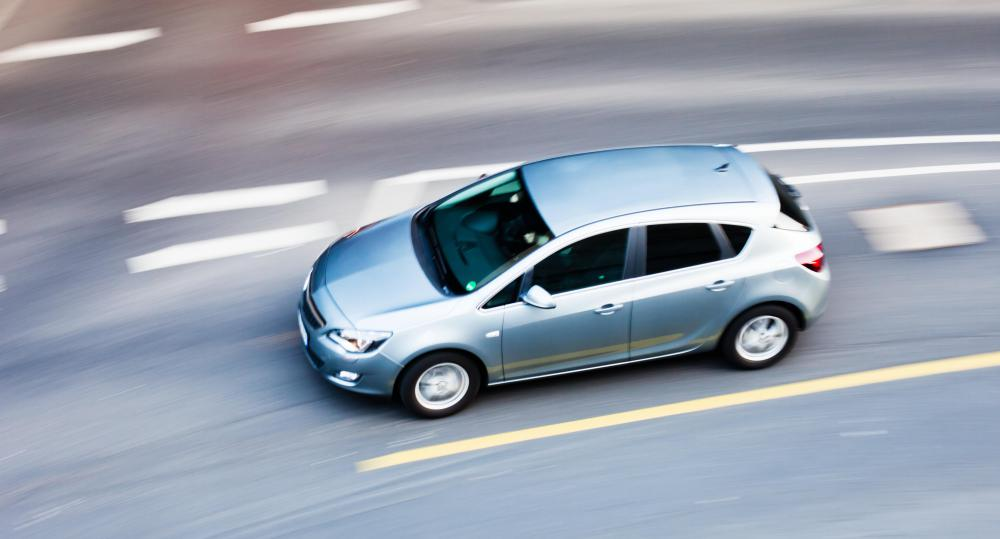# What is Velocity?

C. Martin
C. MartinUnlike speed, the direction the object is moving is required to measure velocity.

Velocity is the measurement of the rate at which an object changes its position. Although it's often confused with speed, the direction in which the object is moving is required for this measurement. There are many different types of velocity measurements, including average, instantaneous, acceleration, radial, and angular. It is a fundamental concept in physics, and has many applications in everyday life.

#### Velocity Versus Speed

The terms speed and velocity are often confused, and in some situations they are used interchangeably. This is technically incorrect, however, because velocity takes an object's displacement — that is, the distance between the starting and ending point — into account and speed does not. If only the distance traveled and time are included, what's being measured is speed.

Speed is a scalar quantity, meaning it is a simple amount of something; it just includes how fast something is moving. In scientific terms, speed is often measured in meters per second, although both the units and the time vary in other applications; a car that's traveling at 60 miles per hour (mph) or 96.5 kilometers per hour (kph), for example, is moving at a specific speed. Only the rate of movement is required — how far the object is moving over a certain amount of time. This is the formula for speed:

Speed = Distance / Time

Velocity is a vector quantity, so it includes not only the amount, but also how it moves in space — the direction. Direction is determined by displacement, which is the distance between where the object started and where it stopped. This is not necessarily equal to the distance traveled; if point A and point B are 2 km apart, but a person walks a 3.5 km path to get from A to B, the displacement is still 2 km. The direction from point A to B must be included when describing this measurement, such as "1.4 meters per second, north."

This is the formula for velocity:

Velocity = Displacement / Time

Because velocity doesn't take the distance traveled into account, an object's average speed and its average velocity can be very different. If a person walks a 3.5 km path from point A to point B in 45 minutes, she has an average speed of 4.66 kph. If the displacement between A and B is only 2 km to the north, however, her average velocity is 2.66 kph, north.

#### Instantaneous and Constant

There are two basic ways to give an object's velocity. Instantaneous velocity is the speed and direction that the object is moving at any given moment, while constant (or average) velocity is when something moves at the same speed and direction over time. For example, a woman may have an instantaneous velocity of 1.4 m/s east at any given point in time when she is walking in that direction. For this to be a constant velocity, she must continue to walk in this direction at this pace for some amount of time. If she changes direction or walks in a circular path that takes her back to her starting point, she does not have a constant velocity because she's not moving in the same direction at the same speed.

#### Acceleration

The term "acceleration" is used to explain how an object changes its velocity over time. In simple terms, the object is speeding up or slowing down, but because this is a vector quantity, the direction must be included as well. An object that changes its direction, even at a constant speed, is changing its acceleration as well. Because velocity is measured in distance over time, such as m/s, acceleration is measured in distance over time over time, or m/s2.

In physics, positive (+) and negative (-) usually indicate direction, not speed; therefore, when something has a negative acceleration, it's not necessarily slowing down, it's moving in a specific direction. Positive is usually right or up, while negative is left or down.

#### Angular Velocity

When an object moves toward or away from its point of origin, it is said to have radial or linear velocity. Angular velocity is when an object is rotating around a center point in a circular motion. Like other types, it is measured by how far the object has traveled over time. Since the object is moving in a circular path, the angular distance can be measured in radians, degrees, or revolutions. Although the object moving in a circle may return to its starting point, it does have direction — clockwise (negative) or counterclockwise (positive).

## You might also Likescienceguy34

@cinder- Your question seems to be: why is it important to get information about the direction an object is moving in as well as its speed?

In short, information about direction can be used to make calculations that would otherwise be impossible.

Consider this problem: A 1 kg box is floating in free space. Two uniform forces of 10 Newtons (remember, force is a vector quantity like velocity—it has both magnitude and direction) are applied to this box.

How fast is the box accelerating? You couldn’t possibly answer this question without knowing information about the direction of the applied forces. If the forces were applied in opposite directions, the box would not be accelerating. If the forces were applied in the same direction, the box would be accelerating at 20 meters per second squared (F=ma, where F=20 N and m = 1 kg).

Direction plays a huge role in physics calculations.

cinder

OK, Physics was not a strong point for me. I understand how figuring velocity would require knowing a direction-if that direction is vertical (gravity would have an increasing affect) vs horizontal (steady gravity affect). However I don't understand why a compass direction is required (East/West etc). How does that make a difference? Someone explain in a way a young child could understand lol!